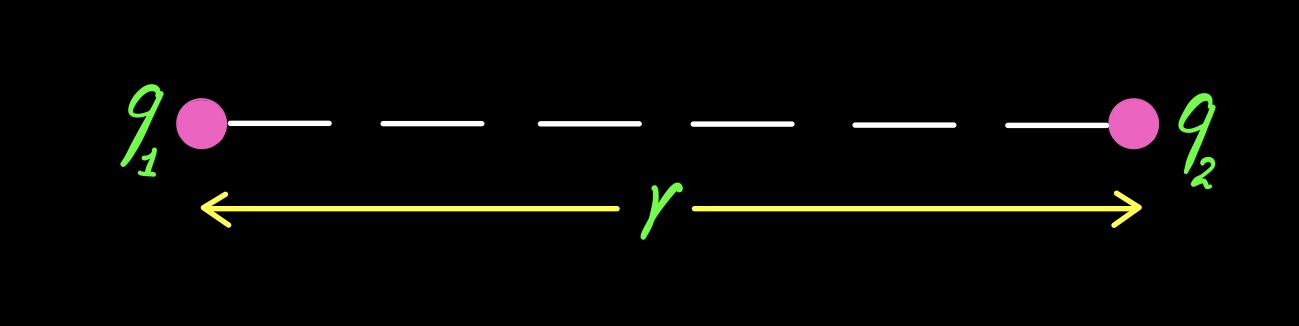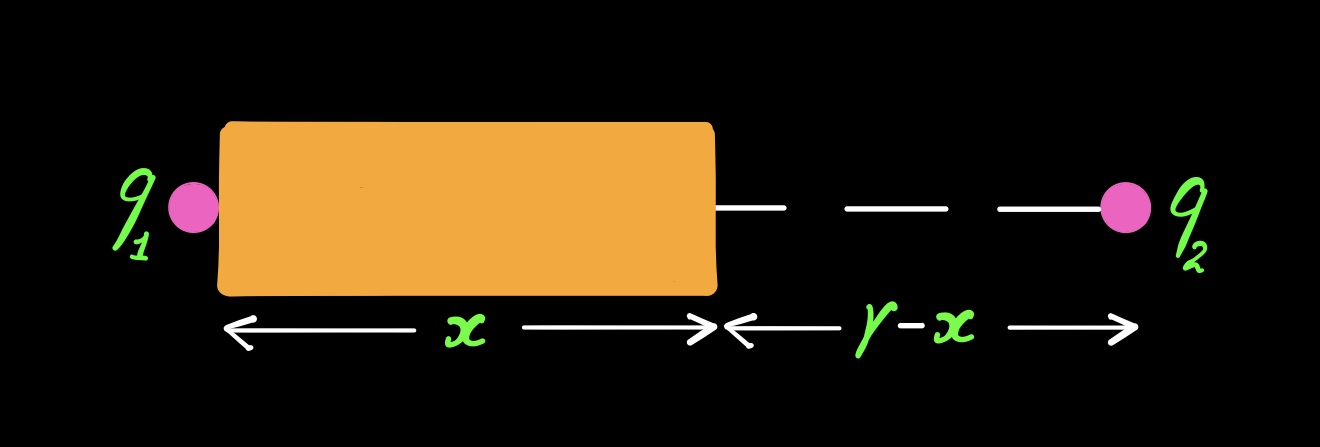# Form of Coulomb’s Law when Dielectric is filled partially between the charges

### Form of Coulomb’s Law when Dielectric is filled partially between the charges

Coulomb’s Law with partially filled Dielectric between the charges :-

Suppose two charge particles q1 and q2 are placed r distance apart in vacuum, then from Coulomb’s Law force acting between them:-$\displaystyle F=\frac{1}{4\pi {{\varepsilon }_{0}}}\frac{{{q}_{1}}{{q}_{2}}}{{{r}^{2}}}$Now let a dielectric medium ( of permittivity εr ) is partially filled between the charges up to a distance x, as shown in figure below:-Now the question is what will be the new value of force between q1 and q2.

For that suppose the whole distance ‘r’ is filled with dielectric medium, then the force becomes:-$\displaystyle F=\frac{1}{4\pi \varepsilon }\frac{{{q}_{1}}{{q}_{2}}}{{{r}^{2}}}=\frac{1}{4\pi {{\varepsilon }_{0}}{{\varepsilon }_{r}}}\frac{{{q}_{1}}{{q}_{2}}}{{{r}^{2}}}=\frac{1}{4\pi {{\varepsilon }_{0}}}\frac{{{q}_{1}}{{q}_{2}}}{{{\left( \sqrt{{{\varepsilon }_{r}}}r \right)}^{2}}}$

Here in the presence of dielectric medium, the distance r, behaves like$\displaystyle \sqrt{{{\varepsilon }_{r}}}r$

So in the given situation, where the dielectric is filled up to the distance x, so we treat distance x as$\displaystyle \sqrt{{{\varepsilon }_{r}}}x$ and the remaining distance as (r-x). Hence the total distance r becomes$\displaystyle [(r-x)+\sqrt{{{\varepsilon }_{r}}}x]$

So the final expression of force becomes:-$\displaystyle F=\frac{1}{4\pi {{\varepsilon }_{0}}}\frac{{{q}_{1}}{{q}_{2}}}{{{[(r-x)+\sqrt{{{\varepsilon }_{r}}}x]}^{2}}}$

Video lecture of above article :-